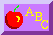# Where Did the Quake Hit?

###### ©1995 The Regents of the University of California
If seismic waves from many miles away shake the ground underneath your feet, can you tell where they came from? Yes, you can tell -- as long as you can read some seismograms.

To do this activity... You need to complete two previous activities: Can You Read a Quake? and Race of the Waves. You need the information you found in both of these activities. You will also need a map of the Pacific Ocean region.

At the end of Race of the Waves, you learned that a seismogram receives Primary (P) waves first, and then it receives Secondary (S) waves. P waves travel through rock faster than S waves. Because of this speed difference, the more time P and S waves have to race away from an earthquake, the farther ahead the P waves will be. In fact, if you measure how many minutes the S waves are behind the P waves, you can estimate how long they have been traveling and how far away they came from. This is how you can locate the epicenter of an earthquake.

Look again at the graph you found in Race of the Waves. You need this graph to answer the following questions.

#### Review: Reading the P and S wave graph

a) Suppose you were 1000 km away from an earthquake.
How many minutes would the P waves need to arrive at your seismograph?
How many minutes would the S waves need to arrive?
How many minutes apart are the P waves and S waves from each other on your seismogram?

b) Suppose you were 2000 km away from an earthquake.
How many minutes would the P waves need to arrive at your seismograph?
How many minutes would the S waves need to arrive?
How many minutes apart are the P waves and S waves from each other on your seismogram?

c) Suppose you were 4000 km away from an earthquake.
How many minutes would the P waves need to arrive at your seismograph?
How many minutes would the S waves need to arrive?
How many minutes apart are the P waves and S waves from each other on your seismogram?

#### Your New Challenge: Locate the Earthquake...

Step 1: Find the time delay.
Do you remember the activity Can You Read a Quake? Go back there for a few minutes and study the seismograms for Tokyo, Sydney, and Hawaii. How many minutes apart are the P and S waves for...

a) Tokyo?
b) Sydney?
c) Hawaii?

In order to continue this activity, you need to write down the minutes for these three locations (the time delay between P and S waves). Here are some hints:

a) Tokyo: The S wave arrived 4.2 minutes after the P wave.
b) Sydney: The S wave arrived almost 6 min after the P wave. (Get a precise answer.)
c) Hawaii: The S wave arrived almost 10 min after the P wave. (Get a precise answer.)

Step 2: Find the distance.
a) You now know how many minutes apart the P waves and the S waves were from each other. Can you tell how far from Tokyo the earthquake actually occurred? Use the P and S wave time graph to find out how many kilometers away Tokyo must have been. Solve it the same way you solved the Review Questions above -- simply work backwards. Instead of starting with the distance (kilometers) and getting a time difference from P to S, use the time difference to get the distance. You will have to guess and check, but be sure to find the best distance that matches the time difference for Tokyo. According to your best estimate, how far from the actual quake was Tokyo? You should get about 3000 or 3100 kilometers.

b) Now determine how far from the quake Sydney and Hawaii were. Use the same method as in step 2 above, but be sure to use the proper time difference that matches each city.

a) Tokyo is approximately 3100 km from the actual quake.
b) Sydney is between 4000 and 5000 km from the actual quake.
c) Hawaii is almost 9000 km from the actual quake.

Step 3: Pinpoint the epicenter on a map.
Here comes the best part: Where was the epicenter of the actual earthquake? To answer this question, you need your three answers from Step 2 above. You also need a map of the Pacific Ocean that has a scale in kilometers.

a) On this map, locate and label Tokyo, Japan. Remember that Tokyo was 3100 km from the actual quake. On your map, how far is 3100 km? Study the map scale and find out how long a line you need to draw on the map to equal1000 km. Triple this line and you have 3000 km. On the map, measure 3100 km away from Tokyo. Now use this distance to draw a circle around Tokyo that has a 3100 kilometer radius.

b) Now draw a second circle around Sydney. Make this second circle big enough to represent the distance from Sydney to the epicenter. Again, use the map scale to determine how large to draw the circle.

c) Now draw a third circle around Hawaii. Make this third circle as big as the distance from Hawaii to the epicenter. Use the map scale again.

d) Where do the three circles cross? If they meet very close to one point, you have probably done your job correctly. Can you name the location of this epicenter?Return to the CEA Science Education Home Page